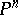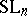Cubic form

(diff) ← Older revision | Latest revision (diff) | Newer revision → (diff)
A homogeneous polynomial of degree 3 in several variables with coefficients in some fixed field or ring. Letbe a field anda cubic form with coefficients in(one calls it a cubic form over). The equationdefines a cubic hypersurface in the projective space, so that the algebraic-geometric theory of cubic forms over an algebraically closed fieldis reduced to the theory of cubic hypersurfaces (see ).
The purely algebraic theory of cubic forms contains, in addition to results concerning the structure of sets of points on cubic hypersurfaces, various results pertaining to the classical theory of invariants. Indeed, the structure of the algebra of (absolute) invariants of a cubic form in two or three variables is known; in these cases the algebra has no syzygies — it is the algebra of polynomials in one (degree 4) and two (degrees 4 and 6) algebraically independent homogeneous generators. If the number of variables exceeds 4, the algebra in question contains syzygies  and its structure is very complicated. H. Poincaré investigated the orbits, stabilizers and canonical representatives, and also the families of orbits under the natural action of the groupon the space of all cubic forms in three and four variables .